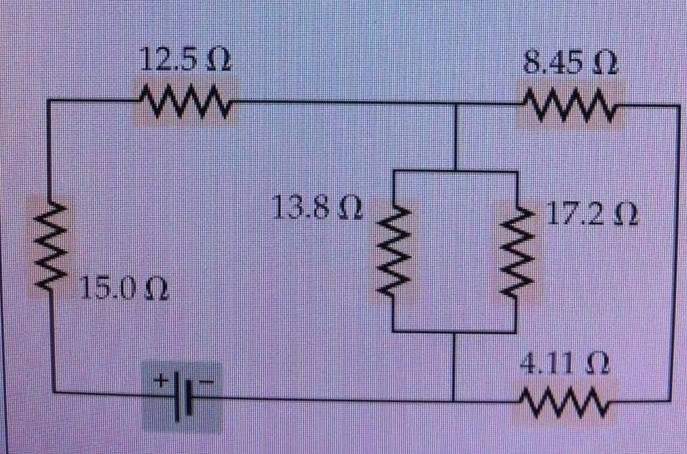# Resistance In Circuits

e334G

## Homework StatementThe current in the 13.8 resistor = .780 A. Goal is to find the current in all resistors.

## Homework Equations

Parallel resistor law - R total = 1 / (1/R1 + 1/R2 + ...)
Series resistor law - R total = R1 + R2 + ...
KCL - sum of currents flowing into a node must be = to sum flowing out
KVL = directed sum of voltages in a closed circuit = 0

## The Attempt at a Solution

Okay, quick preface... a friend randomly asked me if I had any idea how to do this. I haven't been to school in about 6 years, my only knowledge on the subject was what I taught myself way back then and I'm just interested in how this works for personal understanding as I honestly can't recall what's what and what I'm doing wrong and the writeups in Wiki/random tutorial sites are not helping me understand...

First, given the 13.8 resistor with .780 A, I figure out the voltage is 10.764. Applying this to the parallel resistor gives me .626 A. Using the parallel resistor law, I simplify the two to come up with a 7.66 resistor which I name R3. The two currents combined = 1.406 A or I3.

Now I add the other 15 and 12.5 resistors with the serial law for 27.5 or R1. I do the same for 8.45 and 4.11 for 12.56 or R3.
From here, I'm lost...
I call the second half of my current circuit Loop 2 and using KVL, I *think* R3I3 - R2I2 = 0. Going through this formula gives me a current of .858 for I2. Now, with KCL... I2+I3 = I1 = 2.264.

Now when applying KVL to loop 1, R1I1 - R3I3 should = 0, but that's not what I get.
I'm almost positive I'm messing something up when it comes to KVL but I can't tell what and all the examples I've found online haven't helped...

vela
Staff Emeritus
Homework Helper
I didn't check your numbers, but your method sounds correct until your final application of KVL. It's not working because you've ignored the voltage across the battery. You also need to be careful with the signs. The terms for both R1 and R3 should have the same sign based on how you calculated the currents.

Firstly, how do the 8.45- and 4.11-ohm resistors relate, positionally, to the 13.8- and 17.2-ohm resistors?

vk6kro